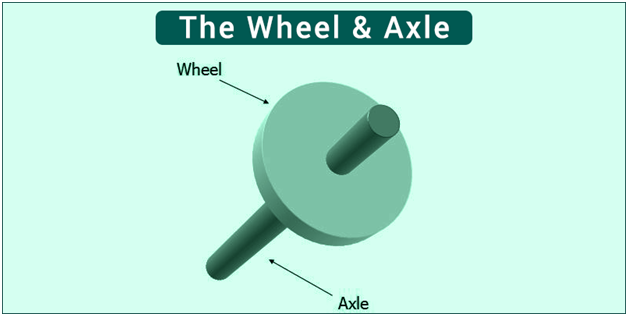# Wheel and AxleObserved as drawings in technological Greek texts, it is one of the first six simple machines identified by the Renaissance scientists. The simple concept of force transformation gives shape to this machine where two segments i.e. a wheel is attached to a smaller axle and their rotations create the force. The rotation is possible due to the bearing or hinge that gives support to the axle. The force applied on one side is amplified by this machine. If at the periphery of a huge wheel a small force is applied, the axle can lift larger loads.

The first use it was put to was for lifting weights. The windlass was an application of this technology where a cylindrical barrel was attached to a pulley. Due to the cylindrical barrel, a mechanical gain is received which can lift heavy loads.

A wheel and axle mechanism can also be viewed as a type of lever. The initial force application is on a tangent on the wheel’s perimeter and the load is managed by the axle with the hinges acting as the fulcrum. If a ratio is computed of the distance of loads from the fulcrum it would give us the mechanical advantage of a wheel and axle. The friction encountered in a moving car is countered by this mechanism.

Two cylinders or disks assembled and rotating in a similar axis and having different diameters result in a wheel and axle. The axle is the slender rod and the force is applied on to the wide object attached to the axle or the wheel. Mechanical advantage is achieved because if at the periphery of a huge wheel a small force is applied, the axle can lift larger loads.

If the mechanism does not store or dissipate energy or does not have elasticity and friction or in short is an ideal machine than the force that will be put on the wheel should equal the output of the power. Force and velocity give us power as their product and thus the force that one applies at the end of the axle should be more than the force one applies at the edge of the wheel. The facts say that the wheel rotates quicker than the axle proving the above-mentioned point.

If the wheel is A and the axle is B we assume that the distance between centre to the edge is a and b. At the edge of the wheel, a force of FA is applied and at the edge of the axle, an output of FB is detected. Thus:

Mechanical Advantage = FB/ FA

Mechanical Advantage = a/b

#### 1 Comment

1. Bhadra Devi Menon

i really loved it thank you very much it was very informative